# Maharashtra Board 12th Maths Solutions Chapter 2 Matrices Ex 2.3

Balbharti 12th Maharashtra State Board Maths Solutions Book Pdf Chapter 2 Matrices Ex 2.3 Questions and Answers.

## Maharashtra State Board 12th Maths Solutions Chapter 2 Matrices Ex 2.3

Question 1.
Solve the following equations by the inversion method.
(i) x + 2y = 2, 2x + 3y = 3
Solution:
The given equations can be written in the matrix form as :
$$\left[\begin{array}{ll} 1 & 2 \\ 2 & 3 \end{array}\right]\left[\begin{array}{l} x \\ y \end{array}\right]$$ = $$\left[\begin{array}{l} 2 \\ 3 \end{array}\right]$$
This is of the form AX = B, where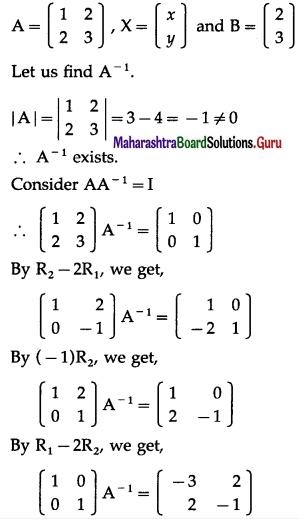∴ A-1 = $$\left[\begin{array}{rr} -3 & 2 \\ 2 & -1 \end{array}\right]$$
Now, premultiply AX = B by A-1, we get,
A-1(AX) = A-1B
∴ (A-1A)X = A-1B
∴ IX = A-1B
∴ X = $$=\left[\begin{array}{rr} -3 & 2 \\ 2 & -1 \end{array}\right]\left[\begin{array}{l} 2 \\ 3 \end{array}\right]$$
∴ $$\left[\begin{array}{l} x \\ y \end{array}\right]$$ = $$=\left[\begin{array}{r} -6+6 \\ 4-3 \end{array}\right]$$ = $$=\left[\begin{array}{l} 0 \\ 1 \end{array}\right]$$
By equality of matrices,
x = 0, y = 1 is the required solution.(ii) x + y = 4, 2x – y = 5
Solution:
x + y = 4, 2x – y = 5
The given equations can be written in the matrix form as:
$$\left[\begin{array}{cc} 1 & 1 \\ 2 & -1 \end{array}\right]\left[\begin{array}{l} x \\ y \end{array}\right]$$ = $$\left[\begin{array}{l} 4 \\ 5 \end{array}\right]$$
This is of the form AX = B ⇒ X ⇒ A-1B
A = $$\left[\begin{array}{cc} 1 & 1 \\ 2 & -1 \end{array}\right]$$
|A| = -1 – 2 = -3 ≠ 0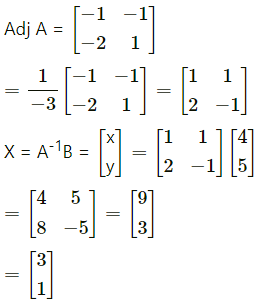By equality of matrices.
x = 3, y = 1

(iii) 2x + 6y = 8, x + 3y = 5
Solution:
The given equations can be written in the matrix form as :
$$\left[\begin{array}{ll} 2 & 6 \\ 1 & 3 \end{array}\right]\left[\begin{array}{l} x \\ y \end{array}\right]=\left[\begin{array}{l} 8 \\ 5 \end{array}\right]$$
This is of the form AX = B, where
A = $$\left[\begin{array}{ll} 2 & 6 \\ 1 & 3 \end{array}\right]$$, X = $$\left[\begin{array}{l} x \\ y \end{array}\right]$$ and B = $$\left[\begin{array}{l} 8 \\ 5 \end{array}\right]$$
Let us find A-1.
|A| = $$\left|\begin{array}{ll} 2 & 6 \\ 1 & 3 \end{array}\right|$$ = 6 – 6 = 0
∴ A-1 does not exist.
Hence, x and y do not exist.Question 2.
Solve the following equations by reduction method.
(i) 2x + y = 5, 3x + 5y = -3
Solution:
The given equations can be written in the matrix form as :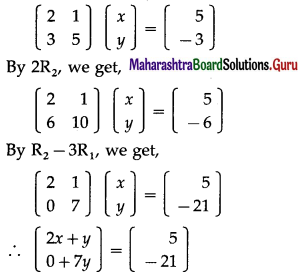By equality of matrices,
2x + y = 5 …(1)
7y = -21 …(2)
From (2), y = -3
Substituting y = -3 in (1), we get,
2x – 3 = 5
∴ 2x = 8 ∴ x = 4
Hence, x = 4, y = -3 is the required solution.

(ii) x + 3y = 2, 3x + 5y = 4.
Solution:
The given equations can be written in the matrix form as :
$$\left[\begin{array}{ll} 1 & 3 \\ 3 & 5 \end{array}\right]\left[\begin{array}{l} x \\ y \end{array}\right]$$ = $$\left[\begin{array}{l} 2 \\ 4 \end{array}\right]$$
By R2 – 3R1, we get
$$\left[\begin{array}{rr} 1 & 3 \\ 0 & -4 \end{array}\right]\left[\begin{array}{l} x \\ y \end{array}\right]$$ = $$\left(\begin{array}{r} 2 \\ -2 \end{array}\right)$$
∴ $$\left[\begin{array}{l} x+3 \\ 0-4 y \end{array}\right]$$ = $$\left[\begin{array}{r} 2 \\ -2 \end{array}\right]$$
By equality of matrices,
x + 3y = 2 …(1)
-4y = -2
From (2), y = $$\frac{1}{2}$$
Substituting y = $$\frac{1}{2}$$ in (1), we get,
x + $$\frac{3}{2}$$ = 2
∴ x = 2 – $$\frac{3}{2}=\frac{1}{2}$$
Hence, x = $$\frac{1}{2}$$, y = $$\frac{1}{2}$$ is the required solution.(iii) 3x – y = 1, 4x + y = 6
Solution:
The given equations can be written in the matrix form as :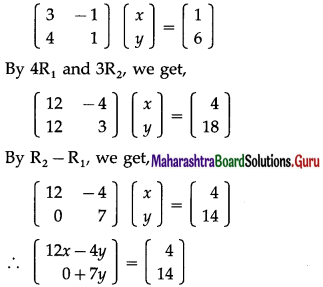By equality of matrices,
12x – 4y = 4 … (1)
7y = 14 … (2)
From (2), y = 2
Substituting y = 2 in (1), we get,
12x – 8 = 4
∴ 12x = 12 ∴ x = 1
Hence, x = 1, y = 2 is the required solution.

(iv) 5x + 2y = 4, 7x + 3y = 5
Solution:
5x + 2y = 4 ………..(1)
7x + 3y = 5 …………(2)
Multiplying Eq. (1) with 7 and Eq. (2) with 5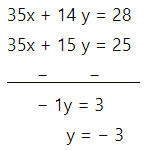Put y = -3 into Eq. (1)
5x + 2y = 4
5x + 2(-3) = 4
5x – 6 = 4
5x = 4 + 6
5x = 10
x = $$\frac{10}{5}$$
x = 2
Hence, x = 2, y = -3 is the required solution.Question 3.
The cost of 4 pencils, 3 pens and 2 erasers is ₹ 60. The cost of 2 pencils, 4 pens and 6 erasers is ₹ 90, whereas the cost of 6 pencils, 2 pens and 3 erasers is ₹ 70. Find the cost of each item by using matrices.
Solution:
Let the cost of 1 pencil, 1 pen and 1 eraser be ₹ x, ₹ y and ₹ z respectively.
Then, from the given conditions,
4x + 3y + 2z = 60
2x + 4y + 6z = 90, i.e., x + 2y + 3z = 45
6x + 2y + 3z = 70
These equations can be written in the matrix form as :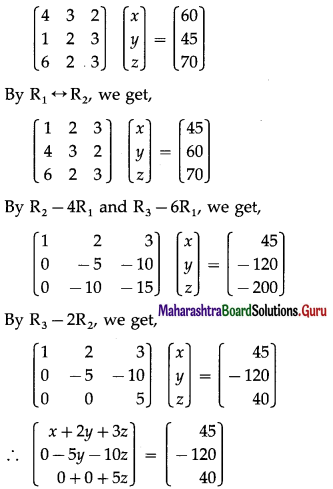By equality of matrices,
x + 2y + 3z = 45 …….(1)
– 5y – 10z = – 120 …….(2)
5z = 40
From (3), z = 8
Substituting z = 8 in (2), we get,
– 5y – 80 = -120
∴ – 5y = -40 ∴ y = 8
Substituting y = 8, z = 8 in (1), we get,
x + 16 + 24 = 45
∴ x + 40 = 45 ∴ x = 5
∴ x = 5, y = 8, z = 8
Hence, the cost is ₹ 5 for a pencil, ₹ 8 for a pen and ₹ 8 for an eraser.Question 4.
If three numbers are added, their sum is 2. If 2 times the second number is subtracted from the sum of first and third numbers, we get 8 and if three times the first number is added to the sum of second and third numbers, we get 4. Find the numbers using matrices.
Solution:
Let the three numbers be x, y and z. According to the given conditions,
x + y + z = 2
x + z – 2y = 8, i.e., x – 2y + 2 = 8
and y + z + 3x = 4, i.e., 3x + y + z = 4
Hence, the system of linear equations is
x + y + z = 2
x – 2y + z = 8
3x + y + z = 4
These equations can be written in the matrix form as :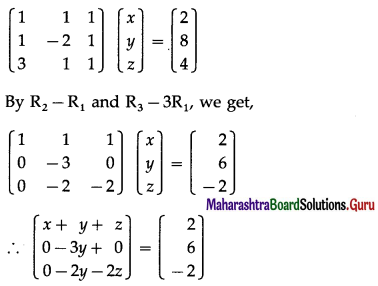By equality of matrices,
x + y + z = 2 ……(1)
-3y = 6 ……(2)
– 2y – 2z = -2 ……..(3)
From (2), y = -2
Substituting y = -2 in (3), we get,
-2(-2) – 2z = -2
∴ -2z = -6 ∴ z = 3
Substituting y = -2, z = 3 in (1), we get,
x – 2 + 3 = 2 ∴ x = 1
Hence, the required numbers are 1, -2 and 3.Question 5.
The total cost of 3 T.V. sets and 2 V.C.R.s is ₹ 35000. The shop-keeper wants profit of ₹ 1000 per television and ₹ 500 per V.C.R. He can sell 2 T. V. sets and 1 V.C.R. and get the total revenue as ₹ 21,500. Find the cost price and the selling price of a T.V. sets and a V.C.R.
Solution:
Let the cost of each T.V. set be ₹ x and each V.C.R. be ₹ y. Then the total cost of 3 T.V. sets and 2 V.C.R.’s is ₹ (3x + 2y) which is given to be ₹ 35,000.
∴ 3x + 2y = 35000
The shopkeeper wants profit of ₹ 1000 per T.V. set and of ₹ 500 per V.C.R.
∴ the selling price of each T.V. set is ₹ (x + 1000) and of each V.C.R. is ₹ (y + 500).
∴ selling price of 2 T.V. set and 1 V.C.R. is
₹ [2(x + 1000) + (y + 500)] which is given to be ₹ 21,500.
∴ 2(x + 1000) + (y + 500) = 21500
∴ 2x + 2000 + y + 500 = 21500
∴ 2x + y = 19000
Hence, the system of linear equations is
3x + 2y = 35000
2x + y = 19000
These equations can be written in the matrix form as :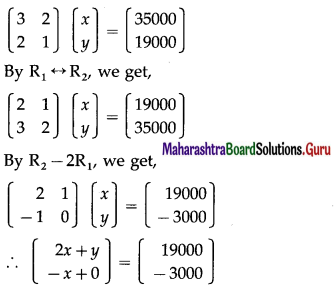By equality of matrices,
2x + y = 19000 ……….(1)
-x = -3000 ……….(2)
From (2), x = 3000
Substituting x = 3000 in (1), we get,
2(3000) + y = 19000
∴ y = 13000
∴ the cost price of one T.V. set is ₹ 3000 and of one V.C.R. is ₹ 13000 and the selling price of one T.V. set is ₹ 4000 and of one V.C.R. is ₹ 13500.